created on 08 Jun 1997 - JLN Labs - Jean-Louis Naudin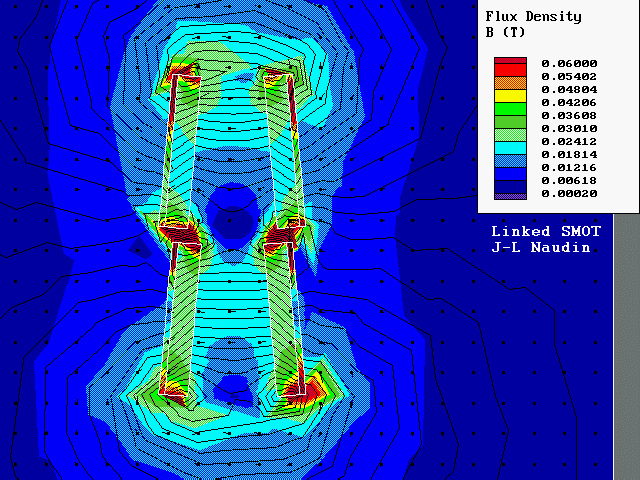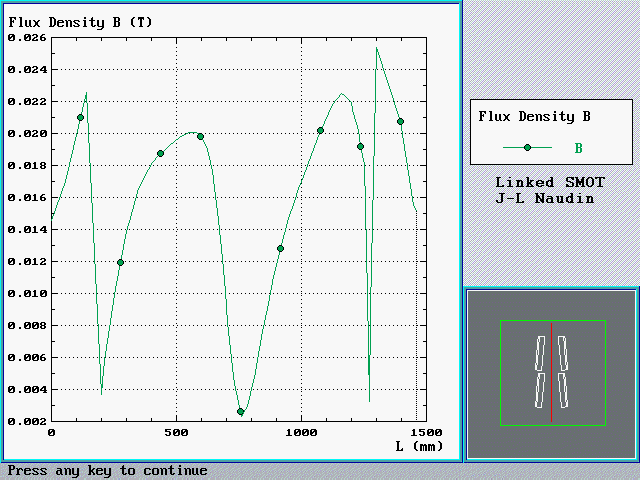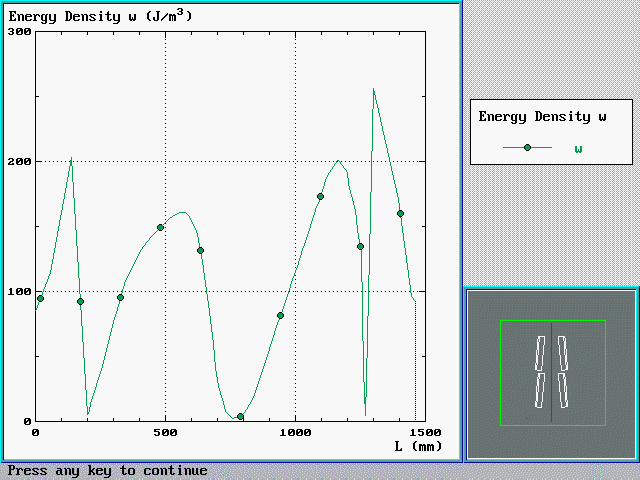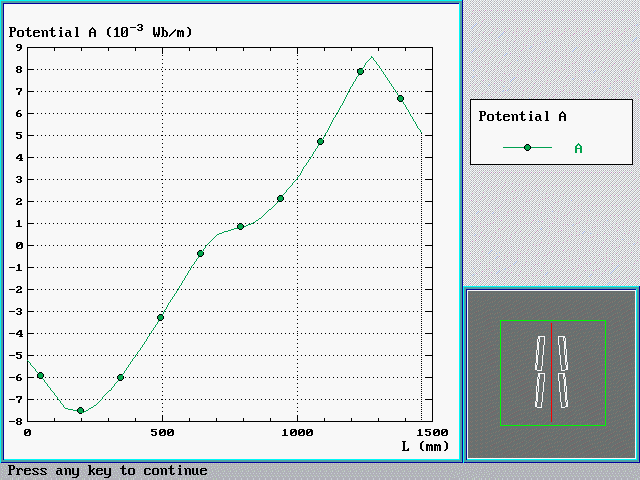Magnet used in simulation : ferrite barium, anisotropic, coer. : 160 kA/m, perm : 1.1 with steel backing.

Magnetic flux density picture : see the BIG blue hole BETWEEN the two ramps, it is bigger than the exit of the second ramp,

Magnetic flux density curve : the curve of the flux density show that the middle blue hole is the reason of the major problems in linking ramps, because of magnetic flux interactions.

Energy density curve: the interesting thing to notice is that the final energy density at the end of the second ramp is BIGGER than at the end of the first ramp, even if this energy density is equal to zero between the two ramp.

This remind to me some experiments about "stepped charged capacitors", the entropy of a system tend to zero if the number of steps tend to infiny ( cf : F. Heinrich "Entropy change when charging a capacitor"

"He showed that when a capacitor in a RC circuit is charged to a final charge in steps, then smaller the voltage steps are, the low energy is dissipated in the resistor. In the limit of infinitesimally small voltages steps, there is no dissipation ( no entropy change )."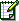Email : JNaudin509@aol.com